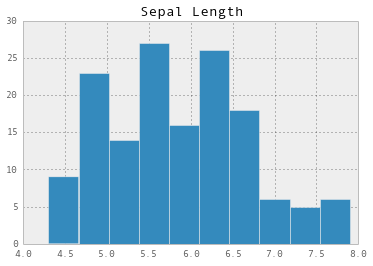Let's look at the classic iris dataset.

First we need tell ipython that we want our plots to show up within the notebook, rather than within a separate window. We can do this with a magic command, which is the name in ipython for a command that starts with a %.

In :
%matplotlib inline


Next we have our import statements. We'll need matplotlib.pyplot for visualization and pandas to use data frames.

In :
import matplotlib.pyplot as plt
import pandas as pd


Now we need to import the iris dataset. In our .py script, this was eight lines of code. With pandas, it's just three!

In :
url = "http://mlr.cs.umass.edu/ml/machine-learning-databases/iris/iris.data"

# I found these headers from looking at the documentation at
# http://mlr.cs.umass.edu/ml/machine-learning-databases/iris/iris.names
headers = ['Sepal Length', 'Sepal Width', 'Petal Length', 'Petal Width', 'Class'
]


Let's see what the data looks like.

In :
iris[:3]

Out:
Sepal Length Sepal Width Petal Length Petal Width Class
0 5.1 3.5 1.4 0.2 Iris-setosa
1 4.9 3.0 1.4 0.2 Iris-setosa
2 4.7 3.2 1.3 0.2 Iris-setosa

3 rows × 5 columns

Now let's plot a histogram for the Sepal Length column.

In :
# I use two brackets around 'Sepal Length' to force pandas to make this
# a data frame rather than just a series, which is like a numpy array.
# The brackets here aren't necessary, but makes printing sepal_lengths
# prettier and makes it easier for us to combine sepal_lengths with other
# data.
sepal_lengths = iris[['Sepal Length']]

# Make the plot pretty!
pd.set_option('display.mpl_style', 'default')

sepal_lengths.hist()

Out:
array([[<matplotlib.axes.AxesSubplot object at 0x107fd6210>]], dtype=object)